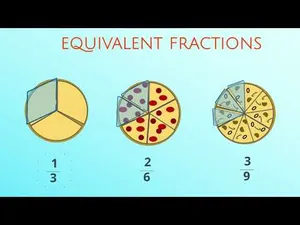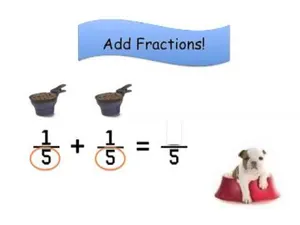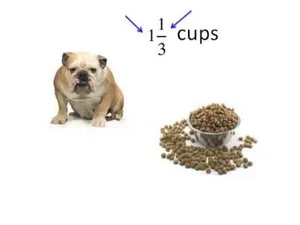# Alien Invasion Equivalent Fractions

4th grade | 4.NF.A.1 | 7 minutes

In this math game, 4th graders practice making equivalent fractions with shaded squares and rectangles to act as scaffolding.

Subject

Category

Unlock this activity and thousands more in eSpark’s playfully personalized learning environment.

Or

Related ActivitiesTry Now
video

Introduction to Equivalent Fractions

Explain Equivalent Fractions

4.NF.A.1Try Now
video

Add & Subtract Fractions with Like Denominators

4.NF.B.3a and 1 moreTry Now
video

Multiplying a Fraction by a Whole Number

Multiply a Fraction and a Number

4.NF.B.4a and 1 moreTry Now
video

Adding & Subtracting Mixed Number Fractions